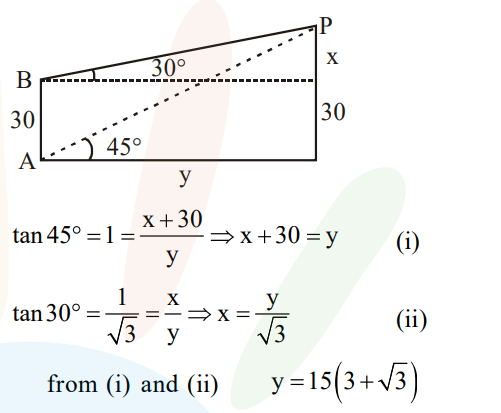# The angle of elevation of the top of vertical tower`
Question:

The angle of elevation of the top of vertical tower standing on a horizontal plane is observed to be $45^{\circ}$ from a point $\mathrm{A}$ on the plane. Let $\mathrm{B}$ be the point 30 $m$ vertically above the point $A$. If the angle of elevation of the top of the tower from $B$ be $30^{\circ}$, then the distance (in m) of the foot of the tower from the point $A$ is:

1. $15(3-\sqrt{3})$

2. $15(3+\sqrt{3})$

3. $15(1+\sqrt{3})$

4. $15(5-\sqrt{3})$

Correct Option: , 2

Solution: